# Question about elastic limit and yield point

songoku
Homework Statement:
This is not homework

Relevant Equations:
None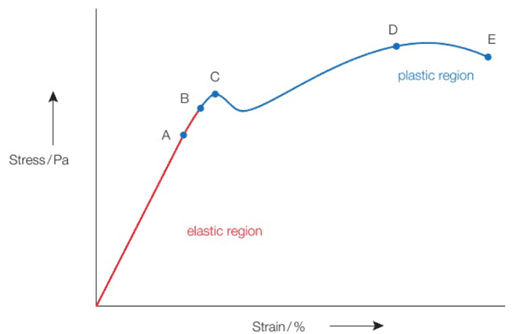Point B is elastic limit and point C is yield point.

The definition given is: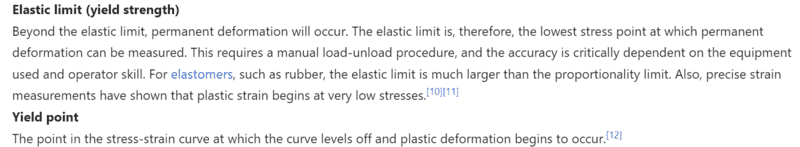Both seems to refer to same definition, it is the point where the elastic deformation ends and plastic deformation begins. But from the graph, the points are different.

And also from this link: https://physics.stackexchange.com/questions/509742/permanent-set-point-vs-elastic-limit

There is graph: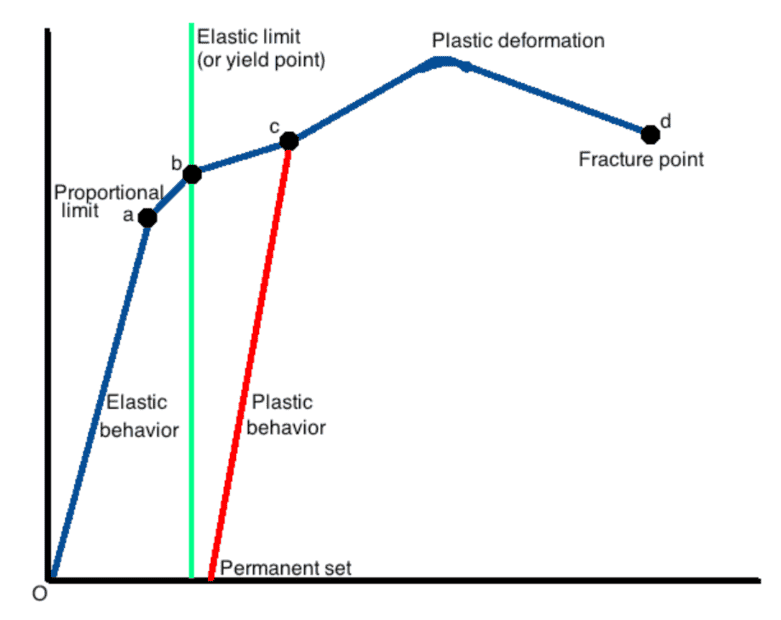In this graph, elastic limit and yield point are one point.

My questions:
Is point of elastic limit the same as yield point?

If no, what is the difference between those two points? And what deformation happens between elastic limit and yield point, is it elastic deformation or plastic deformation?

Thanks

Homework Helper
Gold Member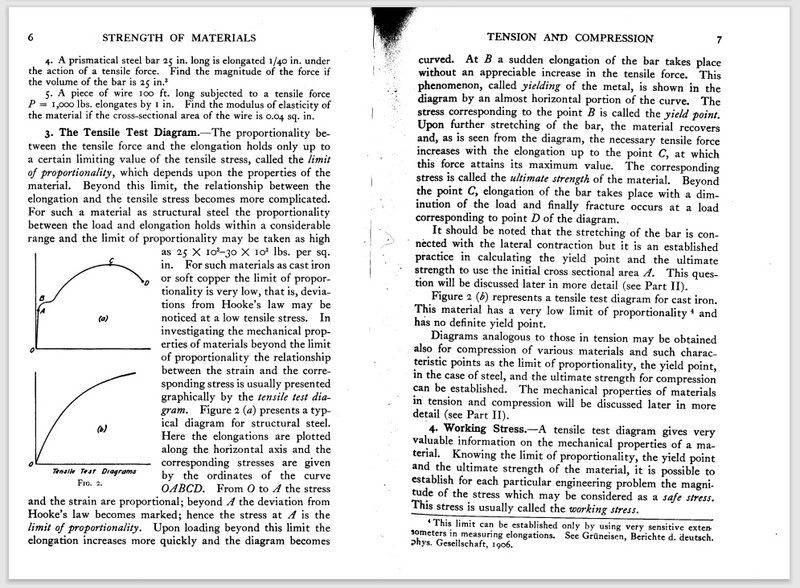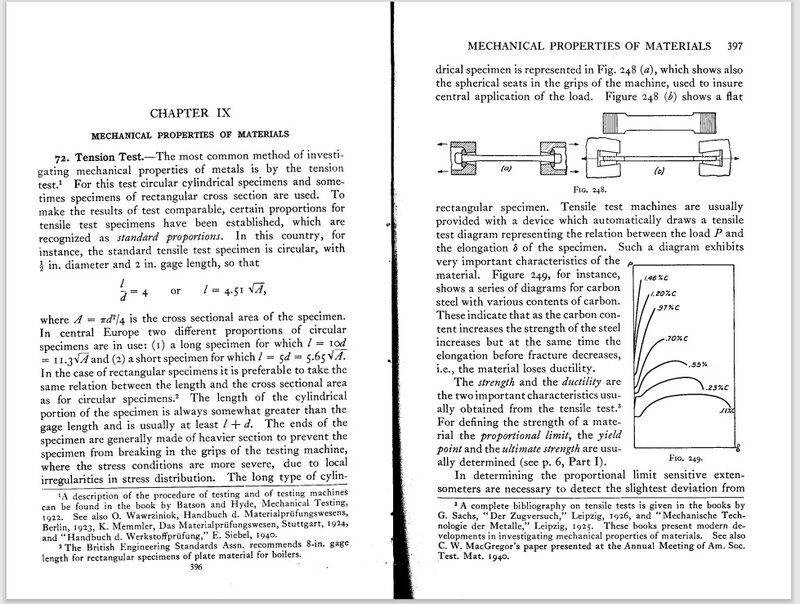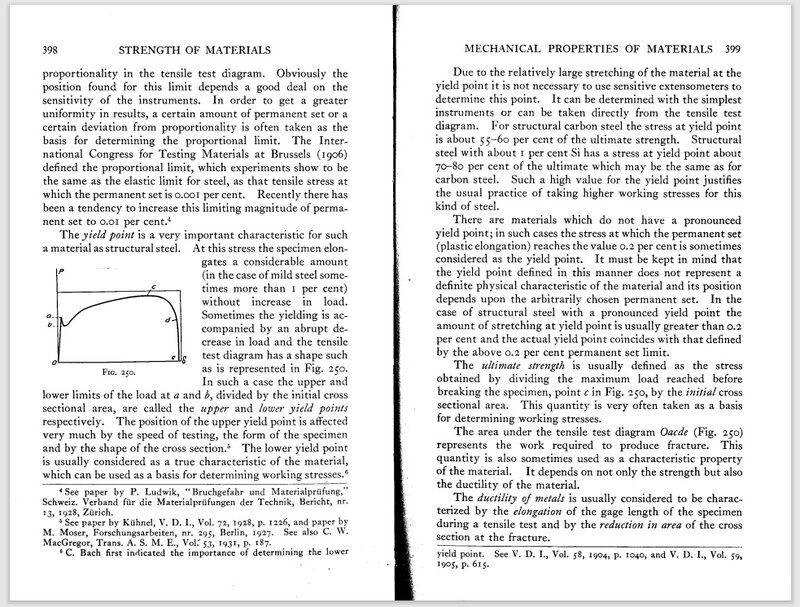Last edited:
•songoku
songoku
Thank you very much Lnewqban

•Lnewqban